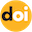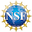Register

### 博客## Research

Here are the latest updates for Changhui Tan's research profile.

Here is the Curriculum Vitae and List of Publications.

### Global regularity for 1D Eulerian dynamics with singular interaction forces

Alexander Kiselev, and Changhui Tan

SIAM Journal on Mathematical Analysis, Volume 50, No 6, pp. 6208–6229 (2018).

Abstract

The Euler–Poisson-alignment (EPA) system appears in mathematical biology and is used to model, in a hydrodynamic limit, a set of agents interacting through mutual attrac- tion/repulsion as well as alignment forces. We consider one-dimensional EPA system with a class of singular alignment terms as well as natural attraction/repulsion terms. The singularity of the alignment kernel produces an interesting effect regularizing the solutions of the equation and leading to global regularity for wide range of initial data. This was recently observed in [Do et al., Arch. Ration. Mech. Anal., 228 (2018), pp. 1–37]. Our goal in this paper is to generalize the result and to incorporate the attractive/repulsive potential. We prove that global regularity persists for these more general models.doi:10.1137/17M1141515Download the Published VersionThis work is supported by NSF grant DMS #1853001

### An asymptotic preserving scheme for kinetic models with singular limit

Alina Chertock, Changhui Tan, and Bokai Yan

Kinetic and Related Models, Volume 11, No 4, pp. 735-756 (2018).

Abstract

We propose a new class of asymptotic preserving schemes to solve kinetic equations with mono-kinetic singular limit. The main idea to deal with the singularity is to transform the equations by appropriate scalings in velocity. In particular, we study two biologically related kinetic systems. We derive the scaling factors, and prove that the rescaled solution does not have a singular limit, under appropriate spatial non-oscillatory assumptions, which can be verified numerically by a newly developed asymptotic preserving scheme. We set up a few numerical experiments to demonstrate the accuracy, stability, efficiency and asymptotic preserving property of the schemes.doi:10.3934/krm.2018030Download the Published Version

### Global regularity for the fractional Euler alignment system

Tam Do, Alexander Kiselev, Lenya Ryzhik, and Changhui Tan

Archive for Rational Mechanics and Analysis, Volume 228, No 1, pp. 1-37 (2018).

Abstract

We study a pressureless Euler system with a non-linear density-dependent alignment term, originating in the Cucker–Smale swarming models. The alignment term is dissipative in the sense that it tends to equilibrate the velocities. Its density dependence is natural: the alignment rate increases in the areas of high density due to species discomfort. The diffusive term has the order of a fractional Laplacian $$(-\partial_{xx})^{\alpha/2}, \alpha\in(0,1)$$. The corresponding Burgers equation with a linear dissipation of this type develops shocks in a finite time. We show that the alignment nonlinearity enhances the dissipation, and the solutions are globally regular for all $$\alpha\in(0,1)$$. To the best of our knowledge, this is the first example of such regularization due to the non-local nonlinear modulation of dissipation.doi:10.1007/s00205-017-1184-2Download the Published Version

### Finite time blow up in the hyperbolic Boussinesq system

Alexander Kiselev, and Changhui Tan

Advances in Mathematics, Volume 325, pp. 34-55 (2018).

Abstract

In recent work of Luo and Hou, a new scenario for finite time blow up in solutions of 3D Euler equation has been proposed. The scenario involves a ring of hyperbolic points of the flow located at the boundary of a cylinder. In this paper, we propose a two dimensional model that we call “hyperbolic Boussinesq system”. This model is designed to provide insight into the hyperbolic point blow up scenario. The model features an incompressible velocity vector field, a simplified Biot–Savart law, and a simplified term modeling buoyancy. We prove that finite time blow up happens for a natural class of initial data.doi:10.1016/j.aim.2017.11.019Download the Published Version

### First-order aggregation models with alignment

Razvan C. Fetecau, Weiran Sun, and Changhui Tan

Physica D: Nonlinear Phenomena, Volume 325, pp. 146-163 (2016).

Abstract

We include alignment interactions in a well-studied first-order attractive–repulsive macroscopic model for aggregation. The distinctive feature of the extended model is that the equation that specifies the velocity in terms of the population density, becomes implicit, and can have non-unique solutions. We investigate the well-posedness of the model and show rigorously how it can be obtained as a macroscopic limit of a second-order kinetic equation. We work within the space of probability measures with compact support and use mass transportation ideas and the characteristic method as essential tools in the analysis. A discretization procedure that parallels the analysis is formulated and implemented numerically in one and two dimensions.doi:10.1016/j.physd.2016.03.011Download the Published Version